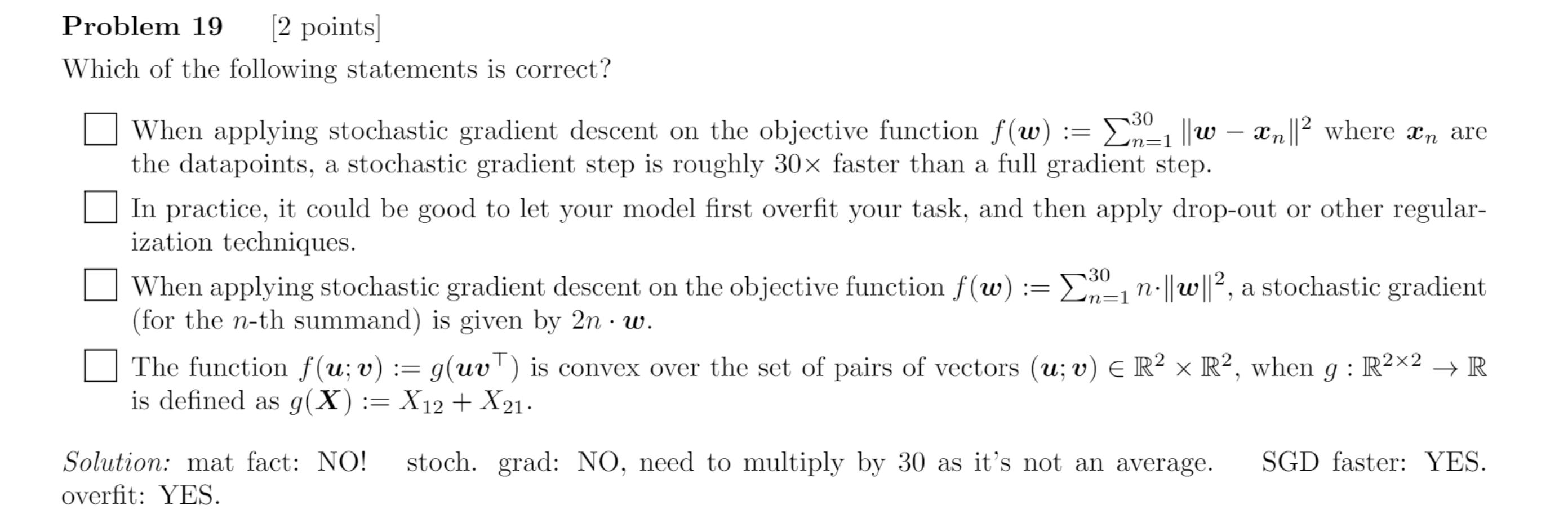### stochastic gradient - problem 19

Hi,

In this question I use the derivative of the individual summand for the stochastic gradient at n. I don't understand why it is wrong, what would be the SGD then in this case? Is it because 1/N is missing in front of the total cost function? If so if we were to rewrite the cost as 1/N(sum(Ln)) does it mean the gradient should be Nn2*w?

Thanks for the help,You should always define the stochastic gradient such that it's expectation = the expectation of the real gradient. Since the loss function here is not an average neither will it's expectation so you need would need to multiply the stochastics gradient in C by 30. As it then would match the expectation of the gradient.

Hope this helps!

If so if we were to rewrite the cost as 1/N(sum(Ln)) does it mean the gradient should be Nn2*w?

No, if you write the cost as 1/N(sum(Ln)), then the gradient is 2n*w

Since you don't have the sum the gradient is N2nw = 302nw, which as @anonymous2 mentioned is in expectation the loss we would get with the full gradient

Page 1 of 1